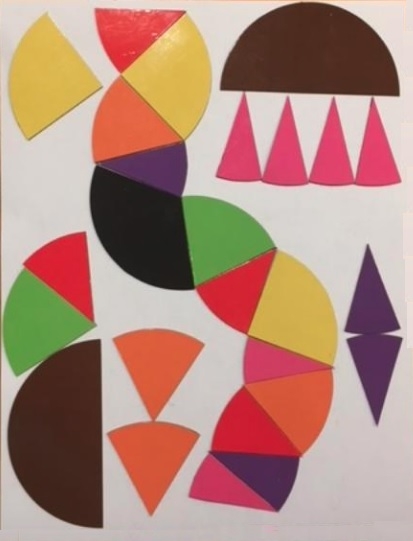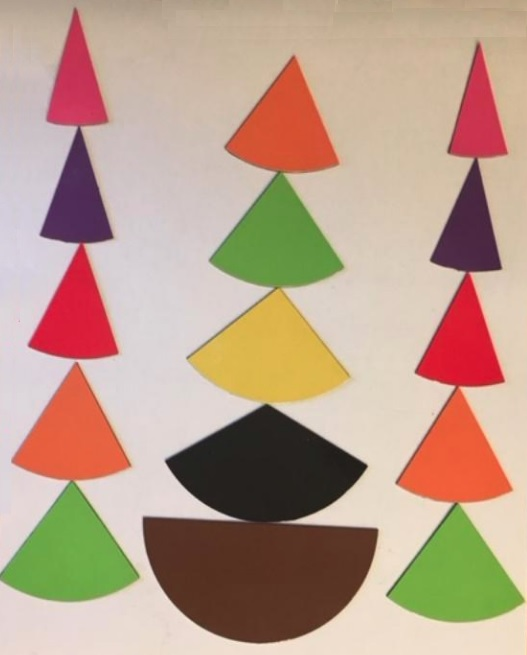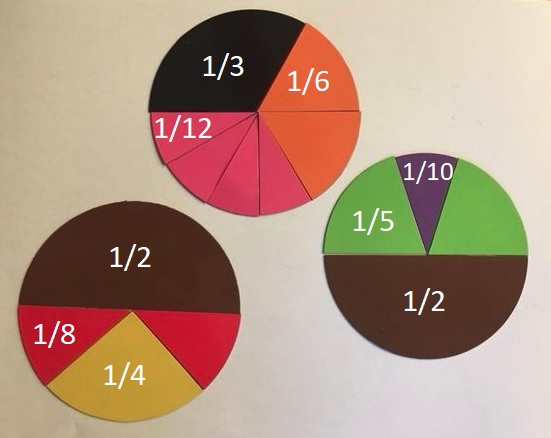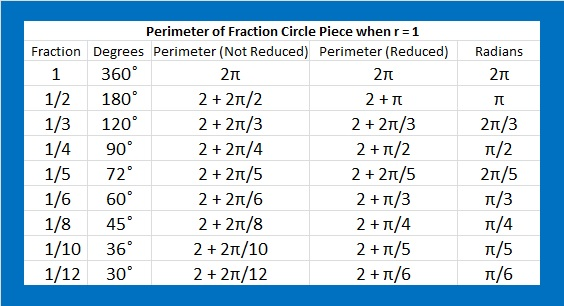# 360 What Can You Do With Fraction Circles?

360 has more factors than any previous number. 240 and 336 held the previous record of 20 factors for each of them. How many factors do you think 360 has? Scroll down to the end of the post to find out.

360 can be evenly divided by every number from one to ten except seven, so it was a good number for the ancients to choose when they divided the circle into 360 degrees.I bought a few fraction circles. Each 51 piece set consists of 1 whole circle as well as circles divided into 2 halves, 3 thirds, 4 fourths, 5 fifths, 6 sixths, 8 eighths, 10 tenths, and 12 twelves. What can you do with fraction circles? You can do a lot with them no matter what your age.

Art and Mathematics

The fraction circle shapes can be used just as tangram shapes to create artwork, big or small. A couple of cool symmetric designs can be found at fraction-art and fraction-circle-art. Adding rectangular fraction pieces will increase the possibilities. Here are some simple artistic designs.Fraction Relationships

You can use fraction circle shapes to explore the relationship between fractions such as ½, ¼, and  ⅟₈;  ⅟₃, ⅟₆  and ⅟₁₂; or ½, ⅟₅ and ⅟₁₀:Areas of Parallelograms, Trapezoids, and CirclesThe picture above shows what happens when the circle is divided into four, six, eight, ten or twelve equal wedges, and the wedges are arranged into something that resembles a parallelogram. This idea can be so easily duplicated with these fraction circles without any cutting.

Here are some good questions to ask:

1. What happens to the top and bottom of the shape when the number of wedges increases?
2. Sometimes the resulting shape will look like a trapezoid, and sometimes it looks more like a parallelogram. Why does that happen?We know that the circumference of any circle is 2πr with π defined as the circumference divided by the radius. π is the same value no matter how big or small the circle is.

We can calculate the area of any of the parallelogram-like shapes or trapezoid-like shapes above. Let’s call the length of the bottom of the shape b₁ and the length of the top b₂. The area of the resulting shape is calculated: A = ½ · (b₁ + b₂) · h. Since b₁ + b₂ = 2πr, and the height equals the radius, we can write our formula for the area of a circle as A = ½ · 2πr · r = πr².

This exercise demonstrates that the area of rectangles, parallelograms, trapezoids, and circles are all related!

Introduction to Pie Charts

Pie charts are a great way to display data when we want to look at percentages of a whole. If you use fraction circles, you are limited to using only to certain percentages, but they can still make a good introduction to the subject. To make the pie chart work either the total of all the degrees will have to equal 360 or the total of all the percents will have to equal 100:After a brief introduction using the fraction circles, try Kids Zone Create a Graph. It’s really easy to use!

Exploring Perimeter and Introducing Radians in Trigonometry

The perimeter of each fraction circle piece can be calculated. If the r = 1, the circumference of the circle is 2π, and we can see an important relationship between the degrees and the perimeter of each piece.What experiences have YOU had with circle fractions? Did you find them frustrating or enlightening? Personally, I like them very much, but I wish they had also been cut into ninths.

Here are some facts about the number 360:

The interior angles of every convex or concave quadrilateral total 360 degrees.

The exterior angles of every convex or concave polygon also total 360 degrees.

Here is all the factoring information about 360:

• 360 is a composite number.
• Prime factorization: 360 = 2 x 2 x 2 x 3 x 3 x 5, which can be written 360 = 2³·3²·5
• The exponents in the prime factorization are 3, 2 and 1. Adding one to each and multiplying we get (3 + 1)(2 + 1)(1 + 1) = 4 x 3 x 2 = 24. Therefore 360 has exactly 24 factors.
• Factors of 360: 1, 2, 3, 4, 5, 6, 8, 9, 10, 12, 15, 18, 20, 24, 30, 36, 40, 45, 60, 72, 90, 120, 180, 360
• Factor pairs: 360 = 1 x 360, 2 x 180, 3 x 120, 4 x 90, 5 x 72, 6 x 60, 8 x 45, 9 x 40, 10 x 36, 12 x 30, 15 x 24 or 18 x 20
• Taking the factor pair with the largest square number factor, we get √360 = (√10)(√36) = 6√10 ≈ 18.974## 3 thoughts on “360 What Can You Do With Fraction Circles?”

1.nerdinthebrain

We have some of those fraction circles (though ours aren’t magnetic, and I am a tad sad now that I can’t use my refrigerator for these things), and I love, love, love them! They come in handy for explaining tons of things (like you said, way beyond the 6th grade), and they’re just fun to play with. 😀

2.abyssbrain

I prefer to use radians instead of degrees for angles especially in calculus.

3.ivasallay

I love radians. There’s no reason to wait until trig or calculus to introduce them. Fraction circles can make them a natural introduction.

This site uses Akismet to reduce spam. Learn how your comment data is processed.